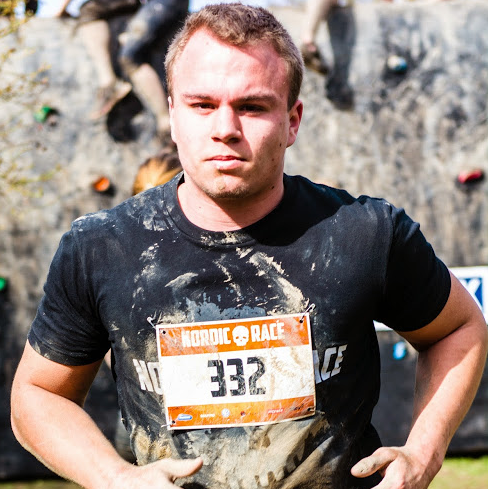# Unity: How to rotate first person camera by dragging touch across?

Maybe its worth noting that I am using the Fingers asset for touch/mouse.

Okay so I have a turret that has a first person camera, on PC it uses the axis Mouse X/Y as I move the mouse around the camera follows. But I need for Mobile to work with the axis Mouse X/Y to drag the camera using touch.

What I need help with is: As my fingers drags across the screen to move the camera's rotation not position. The position is modified by the vehicle.

This is the code I use for PC first person, moving the mouse:

`````` x += Input.GetAxis("Mouse X") * xSpeed * 0.02f;
y -= Input.GetAxis("Mouse Y") * ySpeed * 0.02f;

Quaternion rotation = Quaternion.Euler(y, x, 0f);

``````

How would I use touch dragging to move the rotation? instead of mouse?

Any help with this? Thank you

Here is the current code for touch

``````if (Input.touchCount > 0)
{
if (Input.GetTouch(0).phase == TouchPhase.Moved)
{
Touch touch = Input.GetTouch(0);
x += touch.deltaPosition.x * xSpeed * 0.02f;
y -= touch.deltaPosition.y * ySpeed * 0.02f;

Quaternion rotation = Quaternion.Euler(y, x, 0f);

}
}
``````On

For touch you could use

Input.GetTouch(0).deltaPosition

Try fiddle with something using this:

``````float strength = 2;

if (Input.touchCount == 1) {
if (Input.GetTouch(0).phase == TouchPhase.Moved)
{
Vector2 touchDirection = Input.GetTouch(0).deltaPosition;
// Update ur transform.Rotate the way u desire
// Different depending on using 1st person, 3rd person etc.
}
}
``````